# C Program To Compute Sum Of Geometric Progression

This program receive two numbers and compute the sum of geometric progression. The program implements the geometric progression and output the results when it receives the inputs.

Learn C programming concepts before you start with this example program. Continue reading if you are familiar with the basics.

## Problem Definition

A geometric progression has two conditions.

1.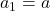where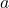is the first term and not equal to 0.
2.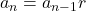for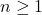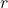is called the common ratio.

\begin{aligned} &Suppose \hspace{2px} a = 1 \hspace{2px} and\hspace{2px}  r = 5, \hspace{2px} then\\ \\&a_1=1\\ &a_2= 5,\\&a_3=25\\& a_4= 125\end{aligned}

Basically, you can compute any term using the following formula.

\begin{aligned}a_n \hspace{1ex} = \hspace{1ex} a \cdot \hspace{1ex} r_{n-1}\end{aligned}

The summation of a geometric progression is given below.

 \begin{aligned} 1\hspace{1ex} + \hspace{1ex} x \hspace{1ex} + \hspace{1ex} x^2\hspace{1ex} + \hspace{1ex} x^3 \hspace{1ex} + \hspace{1ex} x^4 \hspace{1ex} + \hspace{1ex} \cdots \hspace{1ex} + \hspace{1ex} x^{n-1} \hspace{1ex} \cdots\end{aligned}

The summation can be translated into a program for any given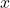value and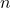value. Such a program is given below.

## Program Source Code

#include <stdio.h>
#include <stdlib.h>
#include <math.h>
int main()
{
int geo_sum, i, x,n;
geo_sum = 1;
printf("Enter the value for x:");
scanf("%d",&x);
printf("Enter the value for n:");
scanf("%d",&n);
if(n <= 0 || x <= 0)
{
printf("Incorrect Value!!\n\n");
}
else
{
printf("The value is valid!!\n\n");
}
for(i = 1;i <= n; i++)
{
geo_sum = geo_sum + pow(x,i);
}
printf("The Sum of Series = %d\n",geo_sum);
system("PAUSE");
return 0;
}

## Output

The input values for the program is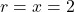and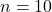. If we use the values in the geometric expression as follows.

1 + 2 + 22 + 23 + 24 + 25 + 26 + 27 + 28 + 29
= 1  + 2 + 4 + 8 + 16 + 32 + 64 + 128 + 256 + 512 + 1024
= 2047

Therefore, the output is correct.

Enter the value for x:2
Enter the value for n:10
The value is valid!!
The Sum of Series = 2047
Press any key to continue . . . _